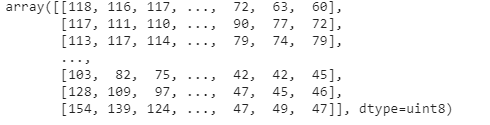# Sampletype float32 in costum script returns UINT8 (sentinel-hub py)

I have used Custom script editor in order to create script that will mask clouds. I want to check if they are really masked by plotting the image.
The problem is that when I use get_data and priint the results, the results are in UINT8.

This is my costum script in the cinfugruation utility:

``````//VERSION=3
function setup() {
return {
input: ["B04", "B08", "CLM"],
output: { bands: 1 },
sampleType:"FLOAT32"
};
}

function evaluatePixel(sample) {
let ndvi = index(sample.B08, sample.B04);
if (sample.CLM == 1) {
return [null]
}
return [ndvi];
}
``````

the idea- return null value if there is a cloud.

Then when I retrieve images and print the values:

``````wms_bands_request = WmsRequest(
data_collection=DataCollection.SENTINEL2_L2A,
data_folder='{}/{}/{}'.format(folder_,date,plot),
bbox=bbox,
time=date,
width=bbox_size,
height=bbox_size,
image_format=MimeType.TIFF,
time_difference=datetime.timedelta(hours=2),#get one image when there is small time differences between them
config=config)

wms_bands_img = wms_bands_request.get_data(save_data=False)
``````I have no idea why does it happen as the sampleType in the costum script is float32.

1 Like

You have defined the “sampleType” outside of the “output” block, so it is essentially ignored, replaced with DEFAULT, which is UINT8.

Try replacing your EVALSCRIPT setup() part with:

``````function setup() {
return {
input: ["B04", "B08", "CLM"],
output: {
bands: 1,
sampleType: "FLOAT32"
}
};
}
``````